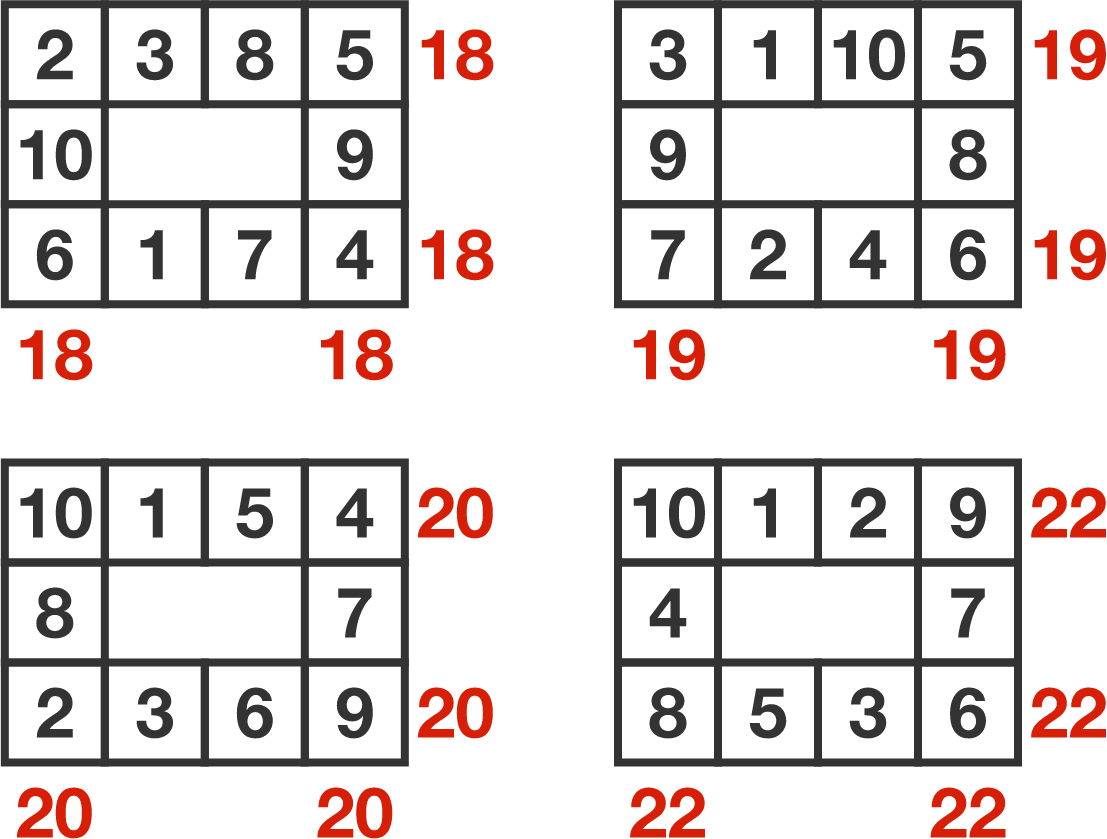# Magic table

Probability Level 1Each of the $3 \times 4$ tables is filled with the numbers 1 through 10 (each used exactly once) so that the sums along each outer row and column are the same number for each table. It is possible to obtain sums of 18, 19, 20, and 22.

Filling out another $3 \times 4$ table in a similar way, is it possible to obtain a sum of 21?

×